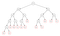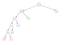# Memoization in a nutshell

In this article, we will look at what memoization is and what problems does it solve with a famous recursive Fibonacci problem in Go.

Fun fact: New Jetpack Compose uses Positional Memoization to prevent ununnecessary calculations when recomposition is happening.

According to Wikipedia:

In computing, memoization or memoisation is an optimization technique used primarily to speed up computer programs by storing the results of expensive function calls and returning the cached result when the same inputs occur again.

Basically with memoization, if we calculated the value of f(x) and we are sure that value of f(x) is always the same for input of x, then we cache the value of f(x) and we won’t recalculate it. That way we can save computation resources. There is a space-time tradeoff but the space complexity of the computation generally is not more than O(n), where time complexity is reduced significantly.

To better understanding we can look at an example. I think nth Fibonacci Number problem is the best way to understand memoization.

According to Wikipedia:

In mathematics, the Fibonacci numbers, form a sequence, called the Fibonacci sequence, such that each number is the sum of the two preceding ones, starting from 0 and 1.

Sequence goes like this: 0,1,1,2,3,5,8,13,21…

That’s a common recursive problem. Because for f(x), first we need to calculate f(x-1) + f(x-2). For f(x-1), we need to calculate f(x-2) + f(x-3) and so on. But this psuedo code doesn’t look like we ever stop. Will we go forever? Obviously not, in recursive calls we need a base case that we can return without going any further.

Let’s look at the explanation of Fibonacci numbers again. Pay attention on the “starting from 0 and 1” part. Because the first two numbers -0 and 1- are fibonacci sequence’s base cases. So we don’t need to calculate f(1) and f(0) as we know their value so we can return from there to last call stack.

The implementation of it should look like this:

This code finds the nth fibonacci number. But it has some performance issues. If you want to calculate nth fibonacci number where n is relatively large, you will see this implementation suffering. Let’s look at why.

We can represent the steps to calculate fib(7) as tree structure:Tree representation of fib(7)

Every node in the tree is sum of its two child nodes except f(2) and f(1) which are the base cases. This is exactly what our function does so far.

Did you see it? The pattern. Even though we are 100% sure fib(3)’s value will never change, we calculated it 5 times! Calculated fib(4)’s value 3 times and fib(5)’s value 2 times. Every time we recalculate those subtrees we do the same computation. It effects our execution time exponentially! The time complexity of this function is O(2^n).

Let’s look at another solution. This time we will cache the values we calculated before. And each time we call fib() again, we will look at the cache. If the value we are looking for exist, we won’t recalculate it.
We will use Golang’s maps to cache the data because time complexity of accessing the data on maps is constant O(1).

The implementation of it should look like this:

The num parameter stays the same, for the cache we pass a map. First we check if we reach the base case. Then we check if fib(x) is already calculated. If so, we get that value and return. If it’s first time to calculate fib(x), we store the result of f(x) to avoid further recalculations.

Side not: Maps in golang are reference type. We don’t need to pass pointer of map. Because by default every recursive call will read/write on the same map.

Let’s look at its tree structure as well as time and space complexity:Tree structure of memoFib(7)

When we calculated fib(3), we cached it in the map. After some time, we faced fib(3) again. But we already calculated it so we don’t need to calculate it again. The same thing goes for fib(4) and fib(5) as I highlighted them with respective colors.

We can save a lot of computation resource and time with memoization. Both time and space complexity of this approach is O(n).

I ran a quick test to see the difference. Both functions calculated the fib(40). I tried to measure execution time with time package in Go but the results are not accurate. Here is the test:

Here is the output:

`6324598663245986It took  493327800  nanoseconds to calculate without memoizationIt took  0  nanoseconds to calculate with memoizatio`

Software Developer @Sahibinden

## More from Teyyihan Aksu

Software Developer @Sahibinden